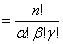# Translations of Encyclopedia about Mathematics

## Combinatory

A combinatory is a subchapter of the calculus of probabilities and a field of arithmetics. It studies the possibility of organising objects in final sets and the possibility of organising under favourable conditions to resolve such tasks as: how many possibilities are there to guess 6 correct numbers out of 49 in a lottery; what combination of letters are possible to create a particular word, etc. Objects can be numbers, letters, persons, attempts and other objects and we usually refer to them as elements. However, when representing a particular task, we usually use numbers or letters. Most types of these kinds of tasks are used for an entertaining form of mathematics. In our case, we will attempt to use theories of numbers and graphs to apply methods of solving problems to certain fundamental samples.

With combinatorial tasks, we usually search the number of possible solutions. Because the number of combinatorial problems is the same as the number of questions, it is possible to only consider some of them in a generalised form. Basic concepts of combinatory are permutation, combination and variation.

1. Permutation

Each set of an unlimited number of elements positioned in some order and in which all elements apply is called a permutation of elements.

1a) Permutation without Repeats

Permutation refers to an order and is derived from the Latin word of permutatio - exchange. Each set of a selected number n of objects is referred to as an n set <n-tící> . A permutation without repeats occurs when each element within it appears only once.

The number of all permutations of n elements is 1.2.3.4…n = n! The attained number n! is called the factorial of n. According to this formula, by replacing <[exchange..] triplets of elements we get 3!, or 6 orderings.

Pn refers to the number of permutations of elements of number n.

Pn  = n . (n-1) . (n-2)….3 . 2 . 1 = n! For the number Pn of all n <n-tic> permutations without repeats, it applies that Pn = n!. However, because n! = n.(n –1)!, it can also be written that Pn = n. Pn -1.  We refer to this calculation as a recursive algorithm.

1b) Permutation with Repeats

If among n elements there are only k different ones and if k<n, we refer to it as permutation with repeats, where each n tici < , more than k elements occur.

A permutation assumes that none of the k elements is lacking in any given n-tici < . If this case can be simplified such that element a occurs in permutation a-times, element b b-times and element c g-times, then the number of all ordered n-tic < and the number of all permutations is

P(n, a,b,c …k)